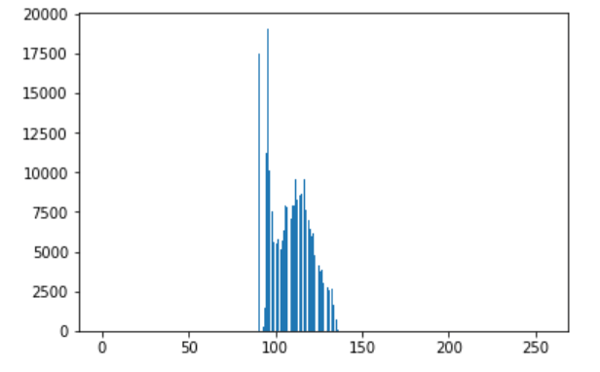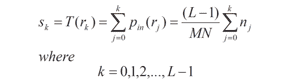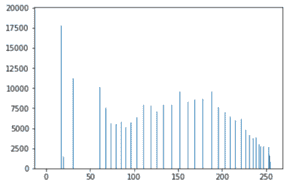Trusted answers to developer questions
Trusted Answers to Developer Questions

Related Tags

python
communitycreator

# What is histogram equalization in Python?

Eman Kashif

Grokking Modern System Design Interview for Engineers & Managers

Ace your System Design Interview and take your career to the next level. Learn to handle the design of applications like Netflix, Quora, Facebook, Uber, and many more in a 45-min interview. Learn the RESHADED framework for architecting web-scale applications by determining requirements, constraints, and assumptions before diving into a step-by-step design process.

Histogram equalization is a process where the intensity values of an image are adjusted to create a higher overall contrast.

Digital Image Processing is a significant aspect of data science. It is used to enhance and modify images so that their attributes are more easily understandable.

Artificial Intelligence also makes use of digital image processing to make for a consistent and advantageous dataset.

Image histograms are largely used to obtain information about image attributes. Image contrast can be determined by looking at the range of pixel intensity values that the histogram bars are spread over.

If the range is low, image contrast is also low. Meanwhile, a higher range of pixel intensity values means a higher contrast.

An example of a low contrast image is shown below.Histogram equalization is done by using the following formula:• L: The maximum intensity level of the image. For a 8-bit image, L is 256.
• M: The width of the image.
• N: The height of the image.
• n: The frequency corresponding to each intensity level.
• rj: The range of values from 0 to L-1.
• pin: The total frequency that corresponds to a specific value of rj.
• rk: The new frequencies.
• sk: The new equalized histogram.

### Code example

#create list of values 0-255pixels=[]for x in range(256):  pixels.append(x)#set width and height of imagewidth,height=img.sizesize=width*heightcounts=[]#for each intensity levelfor i in pixels:  #set counter to 0  temp=0  #traverse through the pixels  for x in range(width):    for y in range(height):      #if pixel intensity is equal to intensity level      #increment counter      if (img.getpixel((x,y))==i):        temp=temp+1    #append frequency of intensity level  counts.append(temp)#initialize list for frequency probabilitiespdf=[]for i in counts:  pdf.append(i/size)#initialize list for cumulative probability cdf=[]total=0for i in pdf:  total=total+i  cdf.append(total)#intialize list for mapping cdftr=[]for i in cdf:  t=round(i*255)  tr.append(t)#initialize list containing new frequencies for equalized hisths=[]for i in pixels:  count=0  tot=0  for j in tr:    if (j==i):      tot=tot+counts[count]    count=count+1  hs.append(tot)#plot equalized histogramplt.bar(pixels,hs)plt.show()

In the above code:

• We start out by storing the frequencies of each pixel intensity in a list: counts.

• Next, we make a list named pdf that stores the probability distribution of each pixel. This is obtained by dividing the frequency of each pixel intensity by the total number of pixels.

• The cdf list is then initialized which stores the cumulative probability of each pixel, found by adding up the previous frequencies.

• The cdf list is then mapped through multiplication with L-1, and stored in tr.

• The new frequencies for each pixel are stored in a list hs, by summing the individual frequencies where the values in tr are equal to the respective pixel intensity.

• Matplotlib is used to plot the equalized histogram.

The equalized histogram will have bars spread out over a larger range of intensity values.

An example is shown below:RELATED TAGS

python
communitycreator

CONTRIBUTOR

Eman Kashif

Grokking Modern System Design Interview for Engineers & Managers

Ace your System Design Interview and take your career to the next level. Learn to handle the design of applications like Netflix, Quora, Facebook, Uber, and many more in a 45-min interview. Learn the RESHADED framework for architecting web-scale applications by determining requirements, constraints, and assumptions before diving into a step-by-step design process.

Keep Exploring

Learn in-demand tech skills in half the time